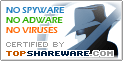# Visual Stats 1.21

SNAPSHOT

Statistics software for data analysis.

Windows AllPlatform :
USD \$120Price :
2.58 MBFile Size :
ScreenshotScreenshot :Popularity :Statistics software for data analysis and multivariate statistical analysis. 1. Probability analysis. 2. Compute descriptive statistics of selected data. 3. Frequency analysis. 4. Compare means and variances. 5. Variance analysis. 6. Univariate linear regression and multivariate linear regression. 7. Linear curve fitting and nonlinear curve fitting. 8. Excel-like data editor is easy to use.